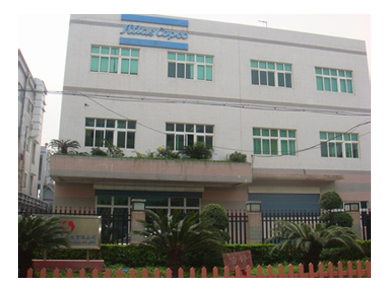首页 >> 新闻资讯 >>行业资讯 >> 空压机选型定律及计算公式

# 空压机选型定律及计算公式

1.波义目定律：假设温度不变则某一定量气体的体积与绝对压力成反比。

V1/V2=P2/P1
2.查理定律：假设压力不变，则气体体积与绝对温度成正比。
V1/V2=T1/T2
3.博伊尔－查理定律
(P1V1)/T1=(T2V2)/T2
P：气体绝对压力
V：气体体积
T：气体绝对温度
4.排气温度计算公式
T2=T1×r(K-1/K)
T1=进气绝对温度
T2=排气绝对温度
r=压缩比(P2/P)P1=进气绝对压力 P2=排气绝对压力
K=Cp/Cv 值空气时K 为1.4(热容比/空气之断热指数)
5.吸入状态风量的计算(即Nm3/min 换算为m3/min)
Nm3/min：是在0℃，1.033kg/c ㎡ absg 状态下之干燥空气量
V1=P0/(P1-Φ1·PD) (T1/T0)×V0 (Nm3/hr dry)
V0=0℃，1.033kg/c ㎡ abs，标准状态之干燥机空气量(Nm3/min dry)
Φa=大气相对湿度
ta=大气空气温度(℃)
T0=273(°K)
P0=1.033(kg/c ㎡ abs)
T1=吸入温度=273＋t(°K)
V1=装机所在地吸入状态所需之风量(m3/hr)
P1：吸入压力=大气压力Pa－吸入管道压降P1 △=1.033kg/c ㎡ abs-0.033kg/c ㎡=1.000kg/c ㎡ abs
φ1=吸入状态空气相对湿度=φa×(P1/P0)=0.968φa
PD=吸入温度的饱和蒸气压kg/c ㎡ Gabs(查表)=查表为mmHg 换算为kg/c ㎡ abs 1kg/c ㎡=0.7355mHg

6.理论马力计算
A 单段式HP/Qm3/min=﹝(P/0.45625)×K/(K-1)﹞×﹝(P2/P1)(K-1)/K-1﹞
B 双段式以上HP/Qm3/min=﹝(P/0.45625)×nK/(K-1)﹞×﹝(P2/P1)(K-1)/nK-1﹞
P1=吸入绝对压力(kg/c ㎡ Gabs)
P2=排气绝对压力(kg/c ㎡ Gabs)
K =Cp/Cv 值空气时K 为1.4
n =压缩段数
HP=理论马力HP
Q=实际排气量m3/min

7.理论功率计算

P1=吸入绝对压力(kg/c ㎡ Gabs)
P2=排气绝对压力(kg/c ㎡ Gabs)
K =Cp/Cv 值空气时K 为1.4
n =压缩段数
KW=理论功率
V=实际排气量m3/min
8.活塞式空压机改变风量之马达皮带轮直径及马力之修正
Dm=Ds×(Qm/Qs)
Ds=马达皮带轮标准尺寸(mm)
Qs=标准实际排气量(m3/min)
Qm=拟要求之排气量(m3/min)
Dm=拟修改之马达皮带轮直径(mm)

7.56m3/min，今假设客户要求提高风量至8.7m3/min，应将马达皮带

Dm=Ds×(Qm/Qs)=400×8.7/7.56=460mm
Nm=Ns×(Qm/Qs)
Nm=修正后之马力数(HP)
Ns=标准之马力数(HP)
Qm、Qs 如上。

Nm=75×8.7/7.56≒86HP
9.空气桶容积之计算
QA=(π/4)×D2×L×0.91
D=空气桶直径(cm)
L=空气桶高度(cm)

Qa=(π/4)×782×152.4×0.91=728.223cm×0.91≒6621
10.现有空压机风量不足，欲维持额定工作压力之风量计算
Qs=Q×(P1+1.033)/(P2+1.033)
Qs=实际状况下系统所需要之空压机之排气量(l/min)
Q=原使用空压机之排气量(l/min)
P1=原使用空压机之工作压力(l/min)
P2=实际状况下系统所需之工作压力(kg/cm2·g)

Os=110×(7+1.033)/(4+1.033)=176 l/min
※即一台空压机若其实际排气量大于176 l/min，即可保证系统压力维持在7 kg/cm2·g。
11.气压缸之空气消耗量计算：
Q=﹝(A1+A2)×L×(P+1)N﹞/1000×e(L/min)
Q=单支气缸之空气消耗量(L/min)
A1=头端活塞有效面积(c ㎡)
A2=杆端活塞有效面积(c ㎡)
L=冲程(cm)
P=使用压力数(kg/c ㎡)
N=每分钟往复数
e=系数1.5－2

100mm，每往复一次)。

Q=0.9×(300/10)×10=270 l/min
270 l/min×1.5(消耗系数)=405 l/min青昊科技有限公司为青昊企业有限公司大陆子公司, 亦为大陆其他公司之总公司, 成立于1998年9月1日,位于深圳宝安沙井西部工业区, 除以上服务项目外, 还增加了给客户做压力容器报装, 报检, 尤其是近几年每个企业都很关注的热能回收, 及节能改造, 能源回收等项目,为很多客户节约能源, 带来高收益回报.

同时大陆其它地方:如东莞,中山,昆山,江门,坪山等地区均设有多个分公司, 并且也是Atlascopco(阿特拉斯.科普柯)之优秀代理商,服务非常方便快捷.

沙井公司目前有4个部门:业务部,工程部,服务部,管理部,共有人员58人, 公司售后服务零件库存: RMB2000万以上(不含大陆其他分部),由原厂商直接供货,还有备用机8台,可随时为客户应急!

各部门业务范围:

业务部: 空压系统设备:空压机,干燥机,精密过滤器,空气储气桶,吸附式干燥机等机器.节能改造评估,热能回收评估,变频改造评估等;

服务部: a.新机试车测试,定期巡检服务. b.一般维修, 紧急维修.c.定期预防保养, 合约保养服务.d.长年限机器大修保养服务.其它品牌也兼做.

工程部: a.工程规划设计,施工与效率评估 .b.配电工程安装兴控制工程提供.c .改善工程评估兴施工服务.

企业宗旨：为客户提供高品质的压缩空气。

企业愿景：致力于打造全球领先视野的行业品牌之一。

企业使命：为客户提供空压系统综合解决方案，满足不同行业类别的需求。

企业文化：诚信、互动、拼搏、创新、务实。

服务优势：拥有领先的各行业需求的空压系统解决方案，完善的销售、售后服务体系，为您的企业降低生产成本，提高生产效率。

- 销售咨询
- 售后客服